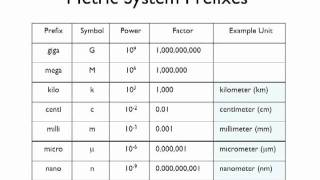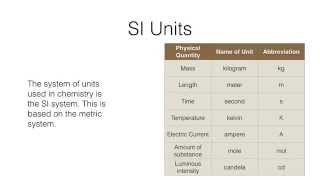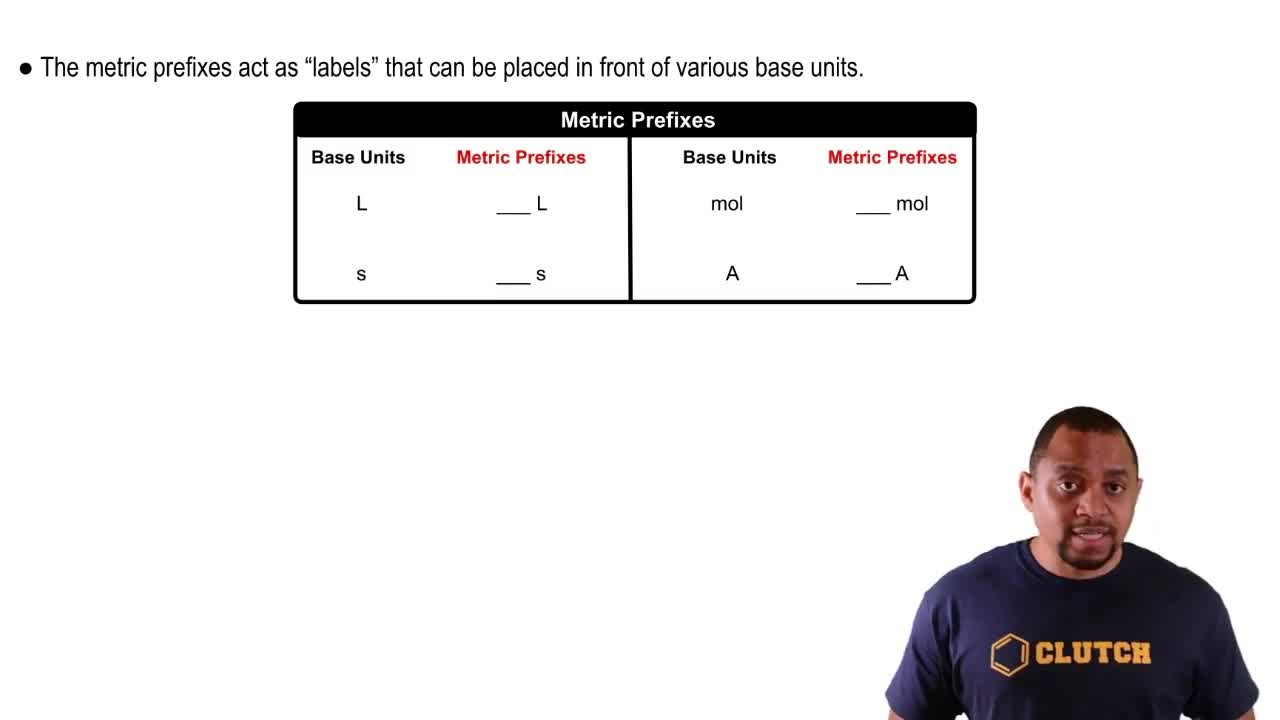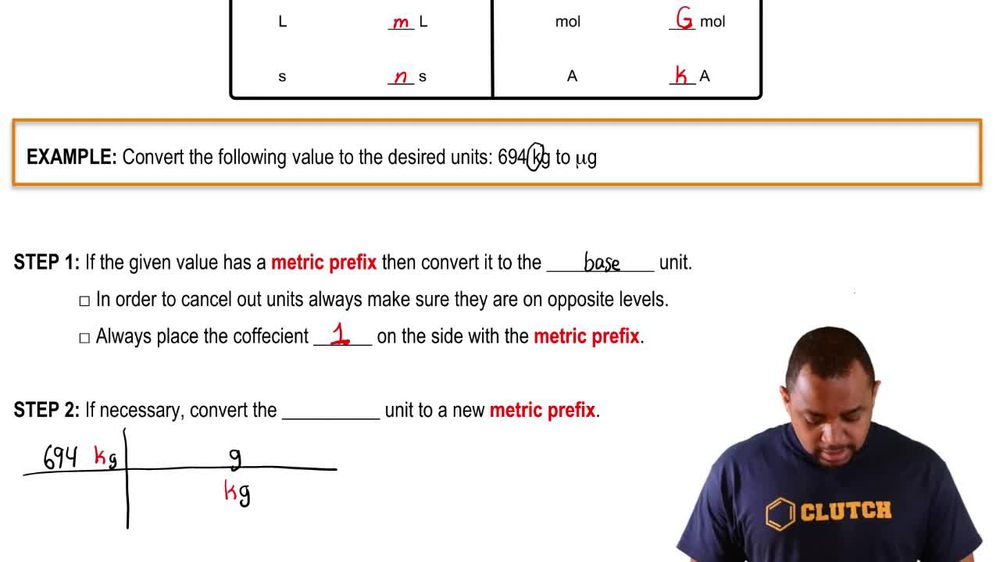Start typing, then use the up and down arrows to select an option from the list.
1. 1. Intro to General Chemistry2. Metric Prefixes
Problem

# A mammalian HELA cell has a diameter of 2 * 10-5 m. Report the diameter of the cell using the most appropriate prefix on the base unit of meter. (LO 1.5) (d) 0.2 mm

Relevant Solution1m
Play a video:
Alright, so we have human here is measured to be 90 microphones or micro meters. What is this measurement? And S. I units? So micrometer is an essay unit, but here we mean we want the measurement in the base unit of meter. Right? So we're just going to be converting micro meter to a meter and we're only going to need one conversion factor for that. So let's start with the number that we have. We have 90 micro meters and we're going to multiply by conversion factor. So micrometers here, we're going to put on the bottom because we want them to cancel out what do we want? We want meters, we're going to put them on top. So micro is a metric prefix and we're always going to put one in front of the metric prefixes and micro is going to be 10 to negative six. Alright, so by doing that, we cancel out the micrometers and now we have just meters left over. So let's go ahead and multiply that number and we get 9. times 10 to the negative five and that's meters. Okay, so that is not one of our answers here. So we just need to go ahead and convert this to um from scientific notation to just regular number. So it is going to obviously have nine in it. You will have nines. Ha ha! Um So we're going to need to move This decimal place to the left because here we have to the -5. So we're going to move this decimal places to the left five times and that will give us an answer of 0.0. So four zeros and then nine meters right? So if you check that 12345, we moved to five decimal places. So it looks like option A. It's going to be our correct answer here. Nice. So A is the right answer choice, right? We're done. Thanks so much for watching.